Filters Date Range Past year (0) Past month (0) Past week (0) Past day (0) Custom range... To   Search Extensions Types Contacts Categories Tag Types Tag Schemes View Results as: JSON ATOM CSV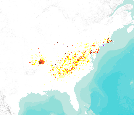This dataset represents actual and predicted suitable habitat for Quercus coccinea (scarlet oak, species code 806) in the Eastern United States as measured by importance value based on data obtained from the Forest Inventory and Analysis (FIA) project, current climate conditions, and future climate projections. This summary unit of this dataset is a 20 by 20 kilometer cell. The actual importance value (IV) was calculated based on the number of stems and basal area of a given tree species relative to other tree species on a plot using about 100,000 FIA plots (representing nearly 3 million tree records) in the 37 states within the United States east of the 100th meridian. These importance values were summarized to...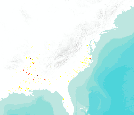This dataset represents actual and predicted suitable habitat for Quercus michauxii (swamp chestnut oak, species code 825) in the Eastern United States as measured by importance value based on data obtained from the Forest Inventory and Analysis (FIA) project, current climate conditions, and future climate projections. This summary unit of this dataset is a 20 by 20 kilometer cell. The actual importance value (IV) was calculated based on the number of stems and basal area of a given tree species relative to other tree species on a plot using about 100,000 FIA plots (representing nearly 3 million tree records) in the 37 states within the United States east of the 100th meridian. These importance values were summarized...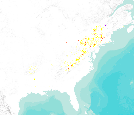This dataset represents actual and predicted suitable habitat for Amelanchier spp. (Serviceberry, species code 356) in the Eastern United States as measured by importance value based on data obtained from the Forest Inventory and Analysis (FIA) project, current climate conditions, and future climate projections. This summary unit of this dataset is a 20 by 20 kilometer cell. The actual importance value (IV) was calculated based on the number of stems and basal area of a given tree species relative to other tree species on a plot using about 100,000 FIA plots (representing nearly 3 million tree records) in the 37 states within the United States east of the 100th meridian. These importance values were summarized to...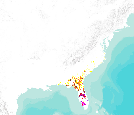This dataset represents actual and predicted suitable habitat for Taxodium distichum var. nutans (pondcypress, species code 222) in the Eastern United States as measured by importance value based on data obtained from the Forest Inventory and Analysis (FIA) project, current climate conditions, and future climate projections. This summary unit of this dataset is a 20 by 20 kilometer cell. The actual importance value (IV) was calculated based on the number of stems and basal area of a given tree species relative to other tree species on a plot using about 100,000 FIA plots (representing nearly 3 million tree records) in the 37 states within the United States east of the 100th meridian. These importance values were...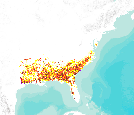This dataset represents actual and predicted suitable habitat for Quercus nigra (water oak, species code 827) in the Eastern United States as measured by importance value based on data obtained from the Forest Inventory and Analysis (FIA) project, current climate conditions, and future climate projections. This summary unit of this dataset is a 20 by 20 kilometer cell. The actual importance value (IV) was calculated based on the number of stems and basal area of a given tree species relative to other tree species on a plot using about 100,000 FIA plots (representing nearly 3 million tree records) in the 37 states within the United States east of the 100th meridian. These importance values were summarized to 20 by...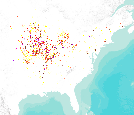This dataset represents actual and predicted suitable habitat for Acer saccharinum (silver maple, species code 317) as measured by importance value based on data obtained from the Forest Inventory and Analysis (FIA) project, current climate conditions, and future climate projections. This summary unit of this dataset is a 20 by 20 kilometer cell. The actual importance value (IV) was calculated based on the number of stems and basal area of a given tree species relative to other tree species on a plot using about 100,000 FIA plots (representing nearly 3 million tree records) in the 37 states within the United States east of the 100th meridian. These importance values were summarized to 20 by 20 kilometer grid cells....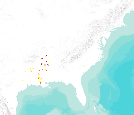This dataset represents actual and predicted suitable habitat for Planera aquatica (water-elm, species code 722) in the Eastern United States as measured by importance value based on data obtained from the Forest Inventory and Analysis (FIA) project, current climate conditions, and future climate projections. This summary unit of this dataset is a 20 by 20 kilometer cell. The actual importance value (IV) was calculated based on the number of stems and basal area of a given tree species relative to other tree species on a plot using about 100,000 FIA plots (representing nearly 3 million tree records) in the 37 states within the United States east of the 100th meridian. These importance values were summarized to 20... This paper describes the potential impacts of warming temperatures and changing precipitation on plants, wildlife, invasive species, pests, and agricultural ecosystems across the multi-state region centered on Chicago, Illinois. We examine a geographic area that captures much of Lake Michigan, including a complex mosaic of urbanization and agriculture surrounding southern Lake Michigan. We consider species currently present within this broad region as well as species that are expected to move into or out of the area as climate zones shift northward through the coming century. Our analysis draws upon disparate data sources to compile projections. We conclude that a complex mixture of land use poses particular challenges... Categories: Publication; Types: Citation; Tags: Agriculture, Climate change, Forest composition, Hardiness zones, Invasive species, All tags...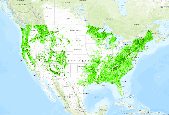Derives forest cover percentage from 33x aggregation of NLCD 2006 classes 41, 42, and 43. Results in log-transformed values representing 0-100% cover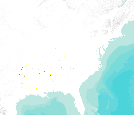This dataset represents actual and predicted suitable habitat for Quercus shumardii (Shumard oak, species code 834) in the Eastern United States as measured by importance value based on data obtained from the Forest Inventory and Analysis (FIA) project, current climate conditions, and future climate projections. This summary unit of this dataset is a 20 by 20 kilometer cell. The actual importance value (IV) was calculated based on the number of stems and basal area of a given tree species relative to other tree species on a plot using about 100,000 FIA plots (representing nearly 3 million tree records) in the 37 states within the United States east of the 100th meridian. These importance values were summarized to...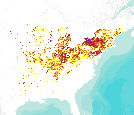This dataset represents actual and predicted suitable habitat for Fraxinus americana (white ash, species code 541) in the Eastern United States as measured by importance value based on data obtained from the Forest Inventory and Analysis (FIA) project, current climate conditions, and future climate projections. This summary unit of this dataset is a 20 by 20 kilometer cell. The actual importance value (IV) was calculated based on the number of stems and basal area of a given tree species relative to other tree species on a plot using about 100,000 FIA plots (representing nearly 3 million tree records) in the 37 states within the United States east of the 100th meridian. These importance values were summarized to...This dataset represents actual and predicted suitable habitat for Quercus laurifolia (laurel oak, species code 820) in the Eastern United States as measured by importance value based on data obtained from the Forest Inventory and Analysis (FIA) project, current climate conditions, and future climate projections. This summary unit of this dataset is a 20 by 20 kilometer cell. The actual importance value (IV) was calculated based on the number of stems and basal area of a given tree species relative to other tree species on a plot using about 100,000 FIA plots (representing nearly 3 million tree records) in the 37 states within the United States east of the 100th meridian. These importance values were summarized to...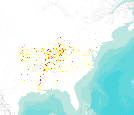This dataset represents actual and predicted suitable habitat for Platanus occidentallis (sycamore, species code 731) in the Eastern United States as measured by importance value based on data obtained from the Forest Inventory and Analysis (FIA) project, current climate conditions, and future climate projections. This summary unit of this dataset is a 20 by 20 kilometer cell. The actual importance value (IV) was calculated based on the number of stems and basal area of a given tree species relative to other tree species on a plot using about 100,000 FIA plots (representing nearly 3 million tree records) in the 37 states within the United States east of the 100th meridian. These importance values were summarized...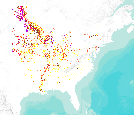This dataset represents actual and predicted suitable habitat for Acer negundo (boxelder, species code 313) in the Eastern United States as measured by importance value based on data obtained from the Forest Inventory and Analysis (FIA) project, current climate conditions, and future climate projections. This summary unit of this dataset is a 20 by 20 kilometer cell. The actual importance value (IV) was calculated based on the number of stems and basal area of a given tree species relative to other tree species on a plot using about 100,000 FIA plots (representing nearly 3 million tree records) in the 37 states within the United States east of the 100th meridian. These importance values were summarized to 20 by...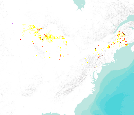This dataset represents actual and predicted suitable habitat for Picea glauca (white spruce, species code 94) in the Eastern United States as measured by importance value based on data obtained from the Forest Inventory and Analysis (FIA) project, current climate conditions, and future climate projections. This summary unit of this dataset is a 20 by 20 kilometer cell. The actual importance value (IV) was calculated based on the number of stems and basal area of a given tree species relative to other tree species on a plot using about 100,000 FIA plots (representing nearly 3 million tree records) in the 37 states within the United States east of the 100th meridian. These importance values were summarized to 20...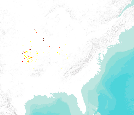This dataset represents actual and predicted suitable habitat for Gymnocladus dioicus (Kentucky coffeetree, species code 571) in the Eastern United States as measured by importance value based on data obtained from the Forest Inventory and Analysis (FIA) project, current climate conditions, and future climate projections. This summary unit of this dataset is a 20 by 20 kilometer cell. The actual importance value (IV) was calculated based on the number of stems and basal area of a given tree species relative to other tree species on a plot using about 100,000 FIA plots (representing nearly 3 million tree records) in the 37 states within the United States east of the 100th meridian. These importance values were summarized...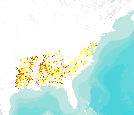This dataset represents actual and predicted suitable habitat for Quercus falcata var.falcata (southern red oak, species code 812) in the Eastern United States as measured by importance value based on data obtained from the Forest Inventory and Analysis (FIA) project, current climate conditions, and future climate projections. This summary unit of this dataset is a 20 by 20 kilometer cell. The actual importance value (IV) was calculated based on the number of stems and basal area of a given tree species relative to other tree species on a plot using about 100,000 FIA plots (representing nearly 3 million tree records) in the 37 states within the United States east of the 100th meridian. These importance values were...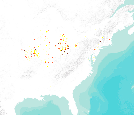This dataset represents actual and predicted suitable habitat for Quercus bicolor (swamp white oak, species code 804) in the Eastern United States as measured by importance value based on data obtained from the Forest Inventory and Analysis (FIA) project, current climate conditions, and future climate projections. This summary unit of this dataset is a 20 by 20 kilometer cell. The actual importance value (IV) was calculated based on the number of stems and basal area of a given tree species relative to other tree species on a plot using about 100,000 FIA plots (representing nearly 3 million tree records) in the 37 states within the United States east of the 100th meridian. These importance values were summarized...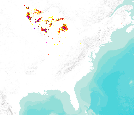This dataset represents actual and predicted suitable habitat for Pinus banksiana (jack pine, species code 105) in the Eastern United States as measured by importance value based on data obtained from the Forest Inventory and Analysis (FIA) project, current climate conditions, and future climate projections. This summary unit of this dataset is a 20 by 20 kilometer cell. The actual importance value (IV) was calculated based on the number of stems and basal area of a given tree species relative to other tree species on a plot using about 100,000 FIA plots (representing nearly 3 million tree records) in the 37 states within the United States east of the 100th meridian. These importance values were summarized to 20... «1...34567